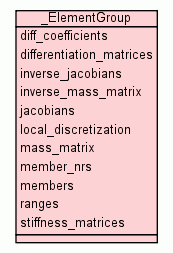[frames] | no frames]

# Class _ElementGroup

source codeOnce fully filled, this structure has the following data members:

 Instance Methods

Inherited from `object`: `__delattr__`, `__getattribute__`, `__hash__`, `__init__`, `__new__`, `__reduce__`, `__reduce_ex__`, `__repr__`, `__setattr__`, `__str__`

 Instance Variables
diff_coefficients
a (d,d)-matrix of coefficient vectors to turn (r,s,t)-differentiation into (x,y,z).
differentiation_matrices
local differentiation matrices D_r, D_s, D_t, i.e.
inverse_jacobians
inverses of jacobians.
inverse_mass_matrix
the element-local inverese mass matrix M^{-1}.
jacobians
list of jacobians over all elements
local_discretization
an instance of hedge.element.Element.
mass_matrix
The element-local mass matrix M.
member_nrs
a list of the element ID numbers in this group.
members
a list of hedge.mesh.Element instances in this group.
ranges
a list of `slice` objects indicating the DOF numbers for each element.
stiffness_matrices
the element-local stiffness matrices M*D_r, M*D_s,....
 Properties

Inherited from `object`: `__class__`

 Instance Variable Details

### differentiation_matrices

local differentiation matrices D_r, D_s, D_t, i.e. differentiation by r, s, t, .....

### ranges

a list of `slice` objects indicating the DOF numbers for each element. Note: This is actually a C++ ElementRanges object.

 Generated by Epydoc 3.0.1 on Sat Aug 29 14:33:24 2009 http://epydoc.sourceforge.net